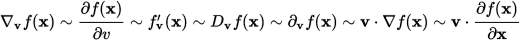# Directional derivative: identity

• I
Hi all,

According to wikipedia:

Directional derivatives can be also denoted by:where v is a parameterization of a curve to which v is tangent and which determines its magnitude.

Can someone explain to me with a mathematical proof the following:

$$\frac {\partial f(x)} {\partial v} = \hat v \cdot \nabla f(x)$$

I don't get this identity except the special example where the partial derivative of f(x) wrt x is a special kind of a directional derivative along the x axis, because the other components of the gradient vector cancel in the dot product with the unit vector along the direction of x.

$$\frac {\partial f(x)} {\partial x} = \hat i \cdot \nabla f(x)$$

So can someone explain to me in a mathematical way how to proof the general identity?

Identity:

$$\frac{\partial f(x)}{\partial v} = \hat v . \nabla f(x)$$

So gradient is:

$$\nabla f(x)= \frac{\partial f(x)}{\partial x} \hat i +\frac{\partial f(x)}{\partial y} \hat j +\frac{\partial f(x)}{\partial z} \hat k$$

Random unit vector is:

$$\hat v = v_x \hat i + v_y \hat j+ v_z \hat k$$

RHS of identity:

$$\hat v . \nabla f(x)= v_x \frac{\partial f(x)}{\partial x} + v_y \frac{\partial f(x) }{\partial y} + v_z \frac{\partial\ f(x) }{\partial z}$$

LHS:

$$\frac{\partial f(x)}{\partial v} = v_x \frac{\partial f(x)}{\partial x} + v_y \frac{\partial f(x) }{\partial y} + v_z \frac{\partial\ f(x) }{\partial z}$$

I don't see it how the partial derivative on the left side is actually a directional derivative.

In plain english: where does the v comes from in $$\frac{\partial f(x)}{\partial v}$$ ?

Last edited:

## Answers and Replies

Stephen Tashi
Science Advisor
For example, think of ##\frac{\partial f(x,y,z)}{\partial((3,6,1))} ## as ## D_t f(x+3t,y+6t,z+1t)|_{t=0} ##.

## = ( \frac{\partial f(x,y,z)}{\partial x})(3) + ( \frac{\partial f(x,y,z)}{\partial y})(6) + ( \frac{\partial f(x,y,z)}{\partial z})(1) ## where the partial derivatives are evaluated at ##(x,y,z)## since after doing the partial derivatives with the varable ##t## present in their arguments, we set ##t = 0##.

It may help to do the work using a specific function like ## f(x,y,z) = x + xy + z^2##.

stevendaryl
Staff Emeritus
Science Advisor
I think they're just saying that these are different notations for the same concept. There is no proof involved in a notation, it's just a convention.

The idea behind a directional derivative in terms of tangents to a parametrized path is just this: Suppose you have a function $f(x,y,z)$ defined at different points in space that returns a real number. Suppose you have a parameterized path giving a location $(x(s), y(s), z(s))$ as a function of a real-valued parameter $s$ that increases as you move along the path. Then you can combine the two to get a function from reals to reals: $F(s) \equiv f(x(s), y(s), z(s))$. Since $F(s)$ is just an ordinary function, you can take an ordinary derivative. Using the chain rule,

$\frac{dF}{ds} = \frac{\partial f}{\partial x} \frac{dx}{ds} + \frac{\partial f}{\partial y} \frac{dy}{ds} + \frac{\partial f}{\partial z} \frac{dz}{ds}$

The right-hand side of the equality can be written more compactly as:

$\frac{dF}{ds} = \vec{V} \cdot \nabla f$

where $\vec{V}$ is the vector with components $V^x = \frac{dx}{ds}, V^y = \frac{dy}{ds}, V^z = \frac{dz}{ds}$, and $\nabla f$ is the "covector" with components $(\nabla f)_x = \frac{\partial f}{\partial x}, (\nabla f)_y = \frac{\partial f}{\partial y}, (\nabla f)_z = \frac{\partial f}{\partial z}$

So the directional derivative $\vec{V} \cdot \nabla$ applied to a scalar field (real-valued function of position, $f$) can be understood as the result of the following computation:
• Find some parametrized path $(x(s), y(s), z(s))$ such that $\vec{V}$ is the corresponding "tangent vector".
• Compute the rate of change of $f$ as $s$ increases along the path.

"I think they're just saying that these are different notations for the same concept. There is no proof involved in a notation, it's just a convention."

No, it is a theorem (though not at all a difficult one), which is in fact proved in the same post that the above quote appears in.

At first, we can speak of the derivative of a function, say

f(x, y, z),​

along a parametrized path

A(s) = (x(s), y(s), z(s)),​

as defined by

d/ds f(A(s))|s=0 = d/ds (f(x(s), y(s), z(s))|s=0 .

At this point, for all we know this depends on more than just the derivative A'(0) at s=0 of the path A(s).

But then by doing the calculation in #3 ("using the chain rule"), we find out that this derivative depends only on A'(0) (and of course on f(x, y, z) near A(0)). So it is the dot product of the gradient ∇f and the tangent vector A'(0) to the path A(s) at s=0.